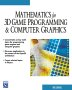# Maths - Multidimensional Algebra and Fields

The geometry of fields is discussed on this page here we discuss how to represent fields using multidimensional algebra types.

## Vector Field

A vector field has a vector value for every point in a space:how this plot was produced.

For instance, if we have a very large mass at the origin, then we can plot the gravitational field, as in the diagram above. Its difficult to find a suitable way to show this, especially in 3 dimensions, an alternative might be to plot lines of constant field force.

## Scalar Field

The above situation can be shown as a scalar field. That on the following diagram the height (y-dimension) shows the scalar value.

We can always get a scalar field from a vector field by using the divergence as shown on this page.how this plot was produced.

 metadata block see also: Correspondence about this page Book Shop - Further reading. Where I can, I have put links to Amazon for books that are relevant to the subject, click on the appropriate country flag to get more details of the book or to buy it from them.Mathematics for 3D game Programming - Includes introduction to Vectors, Matrices, Transforms and Trigonometry. (But no euler angles or quaternions). Also includes ray tracing and some linear & rotational physics also collision detection (but not collision response). Other Math Books Specific to this page here:

This site may have errors. Don't use for critical systems.